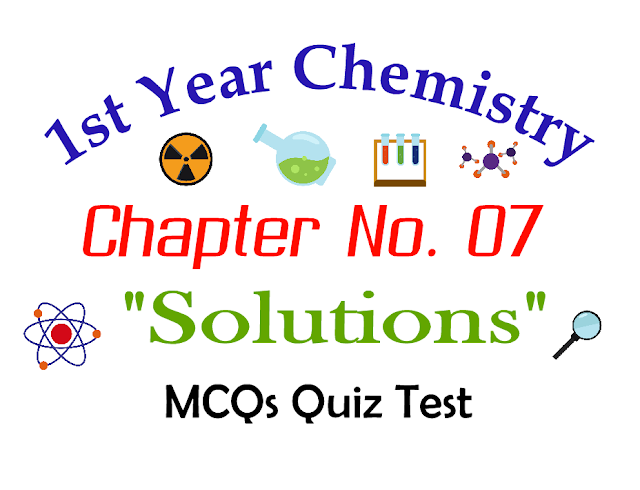# 1st Year Chemistry Chapter 07 Solutions MCQs Quiz Test

If you are looking MCQs for 1st year Chapter Seven "Solutions" MCQs Question Answers with solutions you are here on right website. On this Page you will learn Chapter Seven objective type solved Questions with solutions. All of these chapter wise first Year Chemistry question answers are helpful for upcoming exams and tests preparations. These MCQs are also helpful for entry tests.First Year Chemistry Chapter Seven Notes

You will learn in this Chapter:

• Concentration Units of Solution
• Types of Solution
• Raoult’s Law
• Vapour Pressure of Liquid-Liquid Solutions
• Solubility and Solubility Curves
• Colligative Properties of Solutions
• Energetic of Solution
• Hydration and Hydrolysis

Q.1: 10g NaOH is dissolved in 1000g water. The molality of NaOH is?
1. 1m
2. 0.5m
3. 4m
4. 0.25m
D
Q.2: 250cm3 of 0.2 molar potassium sulphate solution is mixed with 250cm3 of 0.2 molar KCl solution. The molar concentration of K+ ions is?
1. 0.2 molar
2. 0.3 molar
3. 0.25 molar
4. 0.35 molar
B
Q.3: The molality of pure water is?
1. 1
2. 55.5
3. 18
4. 6
B
Q.4: Molal boiling point constant is the ratio of the elevation in the boiling to?
1. Molarity
2. Mole fraction of solvent
3. Molality
4. Mole fraction of the solute
C
Q.5: The number of molecules of sugar in 1dm3 of 1M sugar solution is?
1. 6.02 x 1023
2. 3.42 x 6.02 x 1022
3. 6.02 x 1020
4. None of the above
A
Q.6: Colligative properties can be used for calculating?
1. The density of a compound
2. The lattice energy of a compound
3. The molecular mass of a compound
4. All of the above
C
Q.7: 18g glucose is dissolved in 90g water. The relative lowering of vapor pressure is equal to?
1. 1/5
2. 1/51
3. 5.1
4. 1/55.5
B
Q.8: A solution has 92g ethanol, 96g methanol, and 90g water. Mole fraction of water in the solution is?
1. 0.1
2. 0.4
3. 0.2
4. 0.5
D
Q.9: Elevation of boiling point can be best studied by?
1. Beckmann’s method
2. Landsberger’s method
3. Gibb’s method
4. None of the above
B
Q.10: For observing Colligative properties, which of the following conditions should be fulfilled?
1. The solution should be dilute
2. Solute should be non-electrolyte
3. Solute should be non-volatile
4. All of the above
D
Q.11: When the solvent is containing non-volatile solute particles, then?
1. Vapor pressure is decreased
2. Vapor pressure doesn’t change
3. Vapor pressure is elevated
4. Volume is decrease
A
Q.12: The freezing point is the temperature of the substance at which its solid and liquid phases have the?
1. Different vapor pressure
2. Same volume
3. Same vapor pressure
4. Same density
C
Q.12: A solution of glucose is 10%. The volume in which 1g mole of it will be dissolved is?
1. 1 dm3
2. 200 dm3
3. 1.8 dm3
4. 180 dm3
C
Q.13: Which of the following is not a colligative property?
1. Density
2. Elevation of boiling point
3. Depression in freezing point
4. Osmotic pressure
A
Q.14: The substances which don’t dissolve in a reasonable amount in a particular solvent are-----.?
1. Miscible
2. Well soluble
3. Sparingly soluble
4. Insoluble
C
Q.15: Which one of the following solution of glucose in water is more dilute?
1. 0.1 molar
2. 0.1 molal
3. 1% w/w
4. All have the same concentration
B
Q.15: Which liquid solute is dissolved in the liquid solvent, then the best unit of concentration is?
1. % w/w
2. % w/v
3. % v/v
4. % v/w
C
Q.16: Which of the following is an example of solid in solid solution?
1. Dust particles in smoke
2. Opals
3. Mercury metal in silver metal
4. All of the above
B
Q.17: A colligative property is not represented by?
1. Elevation of boiling point
2. Osmotic pressure
3. Freezing point
4. Relative lowering of vapor pressure
C
Q.18: A solution of KCl in water contains?
1. Free formula units
2. Free ions
3. Free atoms
4. Free molecules
B
Q.19: For very dilute solution the following expression is used?
1. Gram per million
2. Nanogram per million
3. Microgram per million
4. Parts per million
D
Q.20: That solution which possesses a maximum concentration of solute at a given temperature is known as?
1. Unsaturated
2. Saturated
3. Supersaturated
4. Dilute solution
B
Q.21: Which of the following 0.1 m solution will have the lowest freezing point?
1. K2SO4
2. NaCl
3. Glucose
4. Urea
A
Q.22: Which has the highest freezing point?
1. 1.0 m NaCl
2. 1.0 m CaCl2
3. 1.0 m Urea
4. 1.0 m KCl
C
Q.23: Which of the following statement is true about ideal solutions?
1. The change in volume is zero
2. The change in enthalpy is zero
3. The vapor pressure change is zero
4. Both a and b
D
Q.24: The addition of sodium chloride to water, while boiling eggs results to?
1. Decrease boiling point of water
2. Increase the boiling point of water
3. Prevent breaking of eggs
4. Make egg tasty
B
Q.25: Which pair of the following will not form an ideal solution?
1. C2H5Br + C2H5I
2. H2O + C4H9OH
3. C6H14 + C7H16
4. CCl4 + SiCl4
B
Q.26: The study of elevation of boiling point is called?
1. Ebullioscopy
2. Cryoscopy
3. Osmometer
4. None of these
A
Q.27: A solution of glucose is 10% w/v. The volume in which 1g mole of it is dissolved will be?
1. 1dm3
2. 200 cm3
3. 900 cm3
4. 1.8 dm3
D
Q.28: The relative lowering of vapor pressure is equal to?
1. Mole fraction of solvent
2. Mole fraction of solution
3. The molar mass of solute
4. Mole fraction of solute
D
Previous Post
Next Post